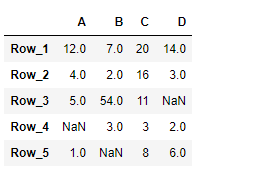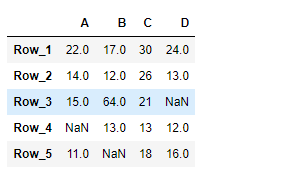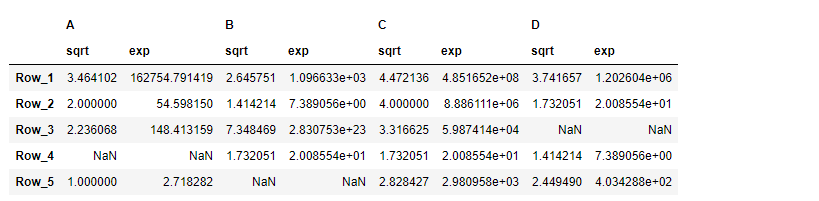# Python | Pandas DataFrame.transform

• Last Updated : 21 Feb, 2019

Pandas DataFrame is a two-dimensional size-mutable, potentially heterogeneous tabular data structure with labeled axes (rows and columns). Arithmetic operations align on both row and column labels. It can be thought of as a dict-like container for Series objects. This is the primary data structure of the Pandas.

Pandas` DataFrame.transform()` function call func on self producing a DataFrame with transformed values and that has the same axis length as self.

Syntax: DataFrame.transform(func, axis=0, *args, **kwargs)

Parameter :
func : Function to use for transforming the data
axis : {0 or ‘index’, 1 or ‘columns’}, default 0
*args : Positional arguments to pass to func.
**kwargs : Keyword arguments to pass to func.

Returns : DataFrame

Example #1 : Use `DataFrame.transform()` function to add 10 to each element in the dataframe.

 `# importing pandas as pd ` `import` `pandas as pd ` ` `  `# Creating the DataFrame ` `df ``=` `pd.DataFrame({``"A"``:[``12``, ``4``, ``5``, ``None``, ``1``],  ` `                   ``"B"``:[``7``, ``2``, ``54``, ``3``, ``None``],  ` `                   ``"C"``:[``20``, ``16``, ``11``, ``3``, ``8``],  ` `                   ``"D"``:[``14``, ``3``, ``None``, ``2``, ``6``]})  ` ` `  `# Create the index ` `index_ ``=` `[``'Row_1'``, ``'Row_2'``, ``'Row_3'``, ``'Row_4'``, ``'Row_5'``] ` ` `  `# Set the index ` `df.index ``=` `index_ ` ` `  `# Print the DataFrame ` `print``(df) `

Output :Now we will use `DataFrame.transform()` function to add 10 to each element of the dataframe.

 `# add 10 to each element of the dataframe ` `result ``=` `df.transform(func ``=` `lambda` `x : x ``+` `10``) ` ` `  `# Print the result ` `print``(result) `

Output :As we can see in the output, the `DataFrame.transform()` function has successfully added 10 to each element of the given Dataframe.

Example #2 : Use `DataFrame.transform()` function to find the square root and the result of euler’s number raised to each element of the dataframe.

 `# importing pandas as pd ` `import` `pandas as pd ` ` `  `# Creating the DataFrame ` `df ``=` `pd.DataFrame({``"A"``:[``12``, ``4``, ``5``, ``None``, ``1``],  ` `                   ``"B"``:[``7``, ``2``, ``54``, ``3``, ``None``],  ` `                   ``"C"``:[``20``, ``16``, ``11``, ``3``, ``8``],  ` `                   ``"D"``:[``14``, ``3``, ``None``, ``2``, ``6``]})  ` ` `  `# Create the index ` `index_ ``=` `[``'Row_1'``, ``'Row_2'``, ``'Row_3'``, ``'Row_4'``, ``'Row_5'``] ` ` `  `# Set the index ` `df.index ``=` `index_ ` ` `  `# Print the DataFrame ` `print``(df) `

Output :Now we will use `DataFrame.transform()` function to find the square root and the result of euler’s number raised to each element of the dataframe.

 `# pass a list of functions ` `result ``=` `df.transform(func ``=` `[``'sqrt'``, ``'exp'``]) ` ` `  `# Print the result ` `print``(result) `

Output :As we can see in the output, the `DataFrame.transform()` function has successfully performed the desired operation on the given dataframe.

My Personal Notes arrow_drop_up
Recommended Articles
Page :